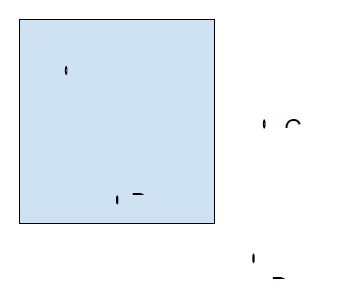# Point Clipping Algorithm in Computer Graphics in C++

Computer graphics deals with drawing images and graphics on a computer screen. Here, we treat the screen as a 2-D coordinate system. This coordinate system starts from top-left (0,0) and ends at bottom-right.

Viewing plane is the area defined for drawing graphics in computer graphics. Or the visible area of the screen.

Clipping is deleting those points or graphics that are outside the viewing plane.

Let's take an example to understand clipping.Here points C and D will be clipped as they are outside the viewing plane marked with blue color.

In order to clip a point in computer graphics. We need to know the coordinates of the viewing place i.e. (Xmin, Ymin) and (Xmax, Ymax). Then we will compare the coordinates of the point with these coordinates.

If (Xmin, Ymin) <= (Xpoint, Y point) <= (Xmax, Ymax), then the point lies inside the viewing plane otherwise it will be clipped off.

## Example

Program to illustrate point clipping,

Live Demo

#include <iostream>
using namespace std;
void pointClipping(int points[], int n, int Xmin, int Ymin, int Xmax, int Ymax) {
cout<<"Points that are removed by Point clipping Algorithm are :"<<endl;
for (int i = 0; i < n; i++){
if ((points[i] < Xmin) || (points[i] > Xmax))
cout<<"("<<points[i]<<","<<points[i]<<")\t";
else if ((points[i] < Ymin) || (points[i] > Ymax))
cout<<"("<<points[i]<<","<<points[i]<<")\t";
}
}
int main() {
int points = {{0, 0}, {-10, 10}, {1000, 1000}, {100, 900}, {501, 311}, {250, 250}};
int Xmin = 0;
int Xmax = 500;
int Ymin = 0;
int Ymax = 500;
pointClipping(points, 6, Xmin, Ymin, Xmax, Ymax);
return 0;
}

## Output

Points that are removed by Point clipping Algorithm are :
(-10,10) (1000,1000) (100,900) (501,311)